Quick Notes Total Entropy

• The total entropy change that occurs in a reaction can be calculated using:• During a chemical reaction, the entropy of reacting particles and their surroundings change.
• How the entropies of the reacting particles change in a reaction is shown by entropy change of the system, ΔSsystem.
• How the entropy of the surroundings changes in a reaction is shown by entropy change of the surroundings, ΔSsurroundings.
• The entropy change of the system and the entropy change of the surroundings can be combined to describe the total entropy change of the reaction, ΔStotal.
• The entropy of the surroundings is linked to the temperature of the system and the enthalpy change that occurs during the reaction.
• It can be calculated by dividing the (-) change in enthalpy (ΔH) by the temperature (T).
• This can be substituted into the total entropy change equation, giving:• Units for enthalpy have to be converted into Joules per mol to match entropy.
• For a reaction to be feasible, the change in total entropy must be positive (+ΔStotal).

Full Notes Total Entropy

This is for Edexcel and Salters A-level Chemistry only. If your course only mentions Free Energy or Gibbs Free Energy, then smile - this page is not for you.

When a chemical reaction happens, it’s not just the entropy of the reacting particles (Ssystem) that changes, but also the entropy of the surroundings (Ssurroundings) of the reaction.

This means the total entropy change that occurs is made up of the entropy change of the reaction system, ΔSsystem and the entropy change of the reactions surroundings, ΔSsurroundings.For a reaction to be feasible, the total entropy change occuring for a reaction must be positive, +ΔS, see below.

## Calculating Total Entropy Change, ΔSTotal

To calculate the total entropy change (ΔStotal) for a reaction, we need to know the entropy change of the system (ΔSsystem) and the entropy change of the surroundings (ΔSsurroundings).

The entropy change of the system (ΔSsystem) can be found using the standard entropies of reactants and products. .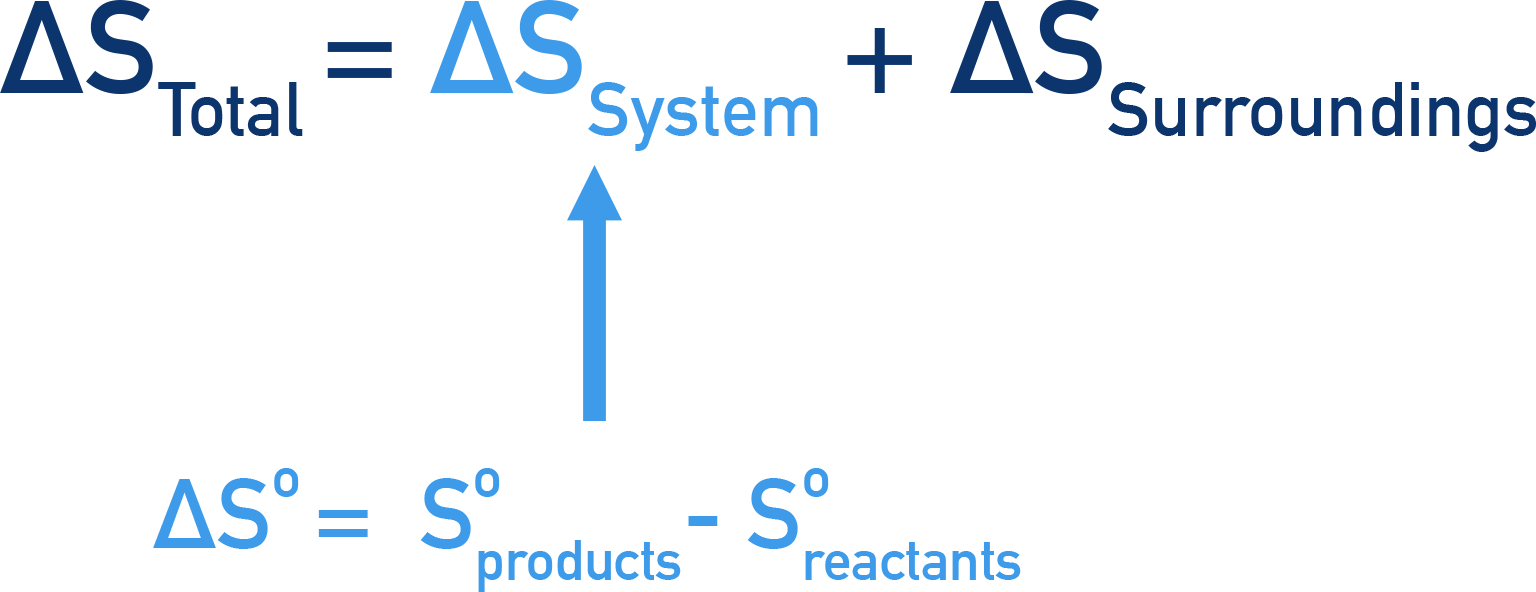The entropy change of surrounding particles is based on the enthalpy change of the reaction.For Example...

In an exothermic reaction (-ΔH), energy is released from a reaction system into its surroundings.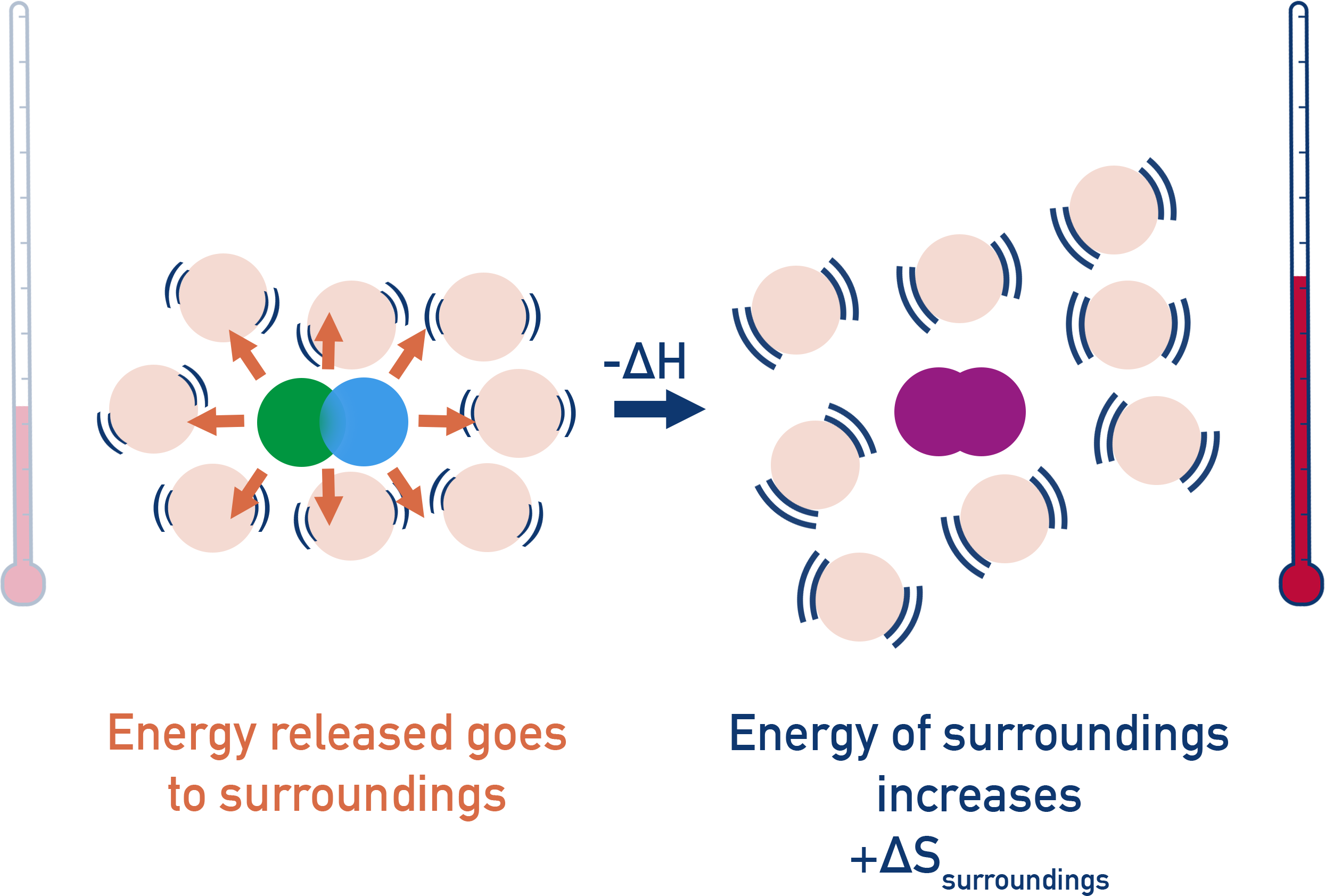This means the surroundings gain thermal energy and its entropy increases (+ΔS).

In an endothermic reaction (+ΔH), energy is taken from a reactions surroundings and goes into the reacting system.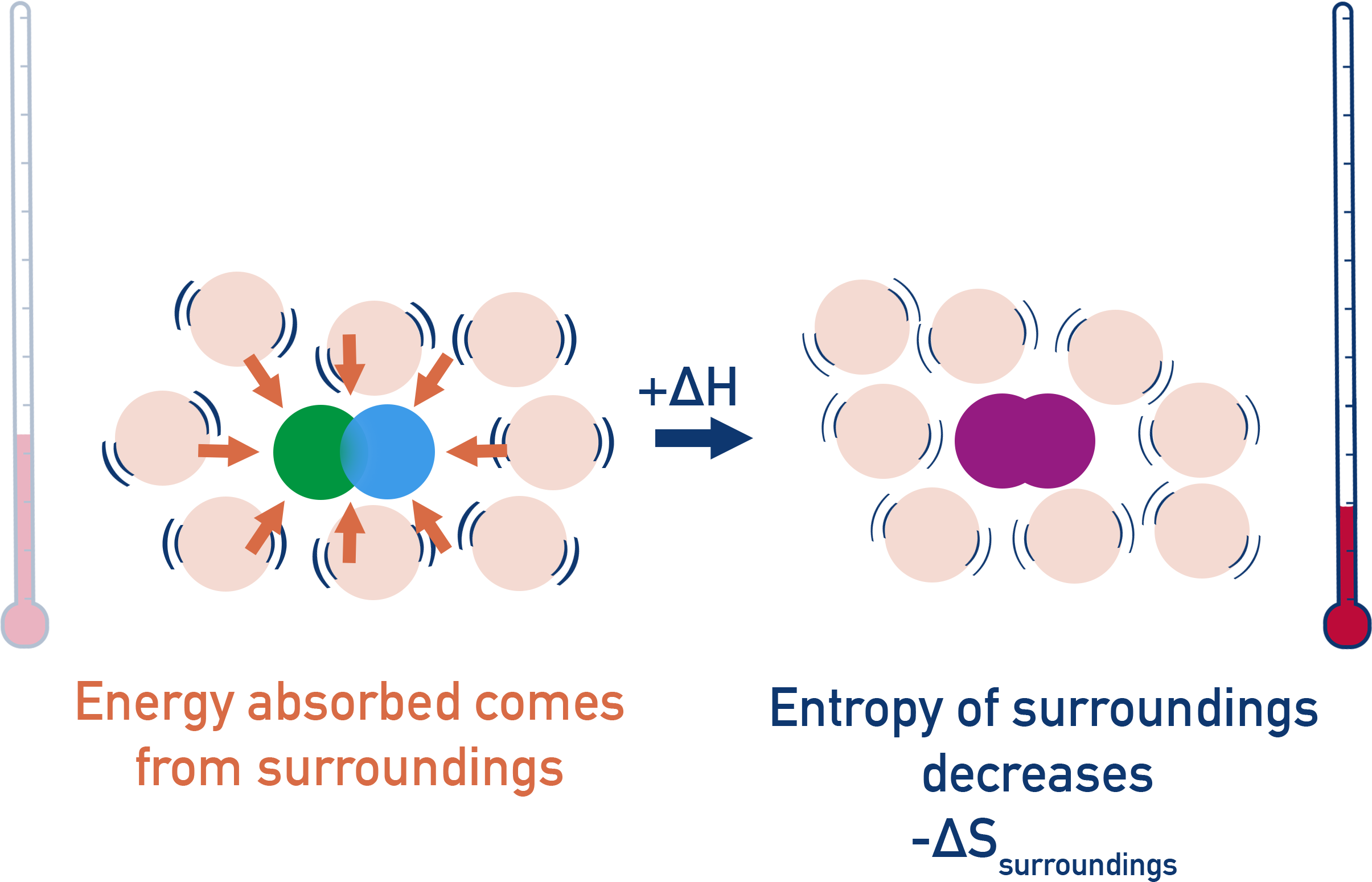This means the surroundings lose thermal energy and its entropy decreases (-ΔS).

As a result, the entropy change of a reaction surroundings, ΔSsurroundings can be found using the enthalpy change of the reaction and the temperature at which the reaction occurs.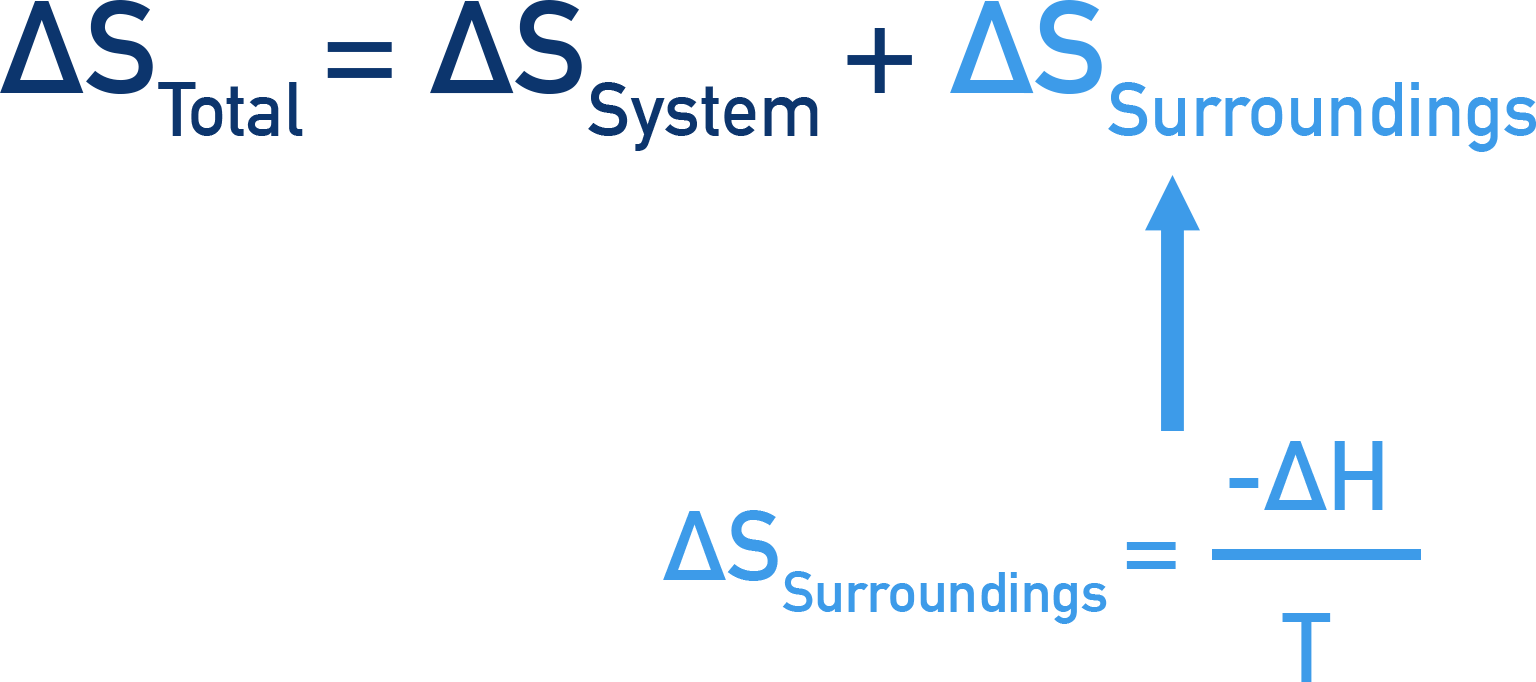We can substitute this form of ΔSsurroundings into the main equation for total entropy change, giving us a new way to calculate total entropy change.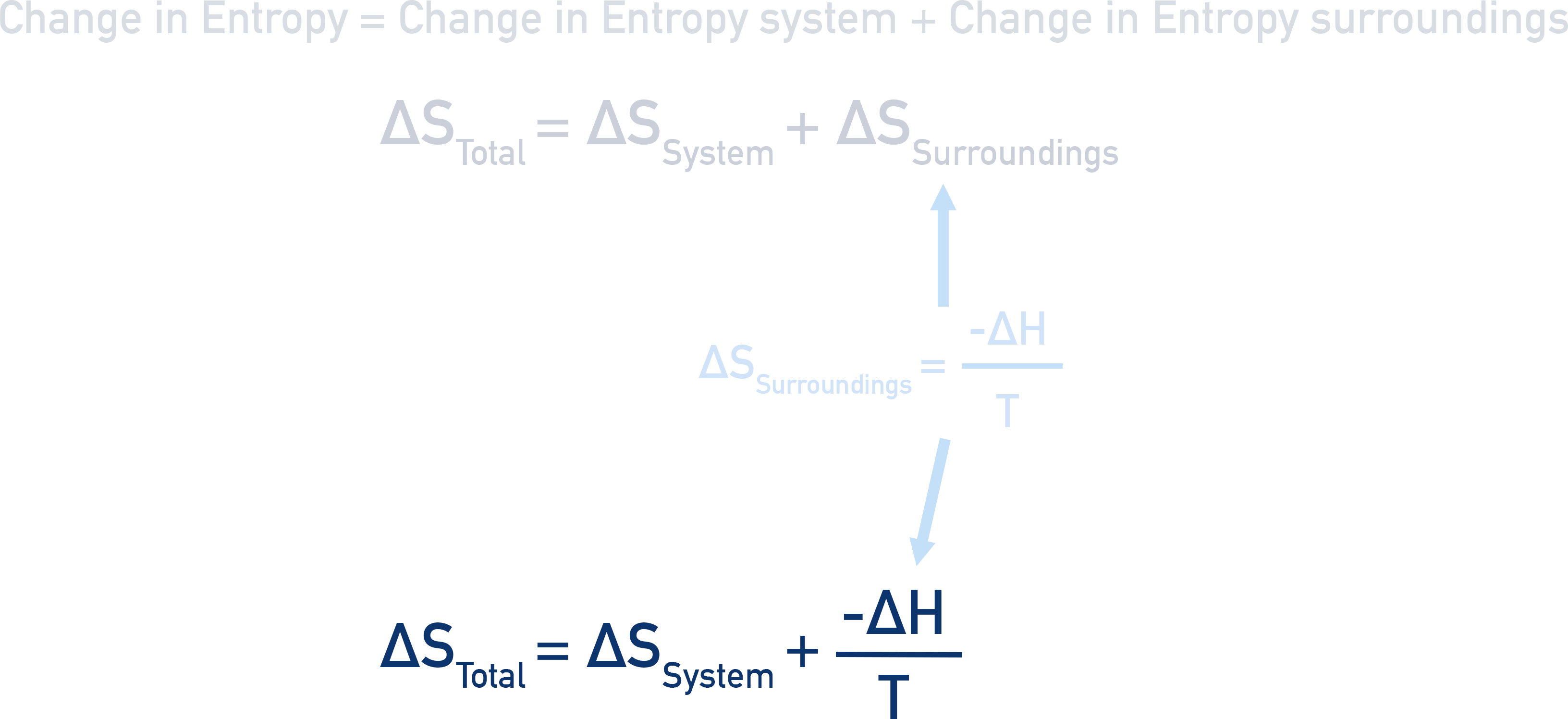When calculating it’s important to remember the units. Entropy is given in J K-1 mol-1 and Enthalpy in kJ mol-1. For calculations, convert enthalpy into J (x 1000).

## Feasibility

For a reaction to be 'allowed' to occur, total entropy needs to increase. If ΔStotal is a positive value, the reaction is feasible and is allowed to happen.

Be careful though, because even if a reaction is allowed to happen, in reality the reaction may still not happen. High activation energy barriers and very slow rates of reaction can stop a reaction from occurring, even if it ‘makes sense’ with regards to the enthalpy and entropy changes.

The terms feasible and spontaneous are often both used at this level to describe reactions that are allowed occur. Used in this way, there is no difference between the two.

## How can reactions with a negative entropy change still be feasible?

In order for a reaction or process to occur, the entropy of the universe has to increase. In a simple sense, this means energy has to end up more spread out in the universe after a reaction has occured compared to before. As entropy basically describes the spreading out (or 'dispersal') of energy, if entropy increases, energy is ending up more spread out or dispersed in the universe.

The universe is a pretty big place! In reality, we can't consider the whole universe when we study reactions. Instead we have to treat the reaction system and its surroundings as a 'mini universe'. A bit like a perfectly sealed box.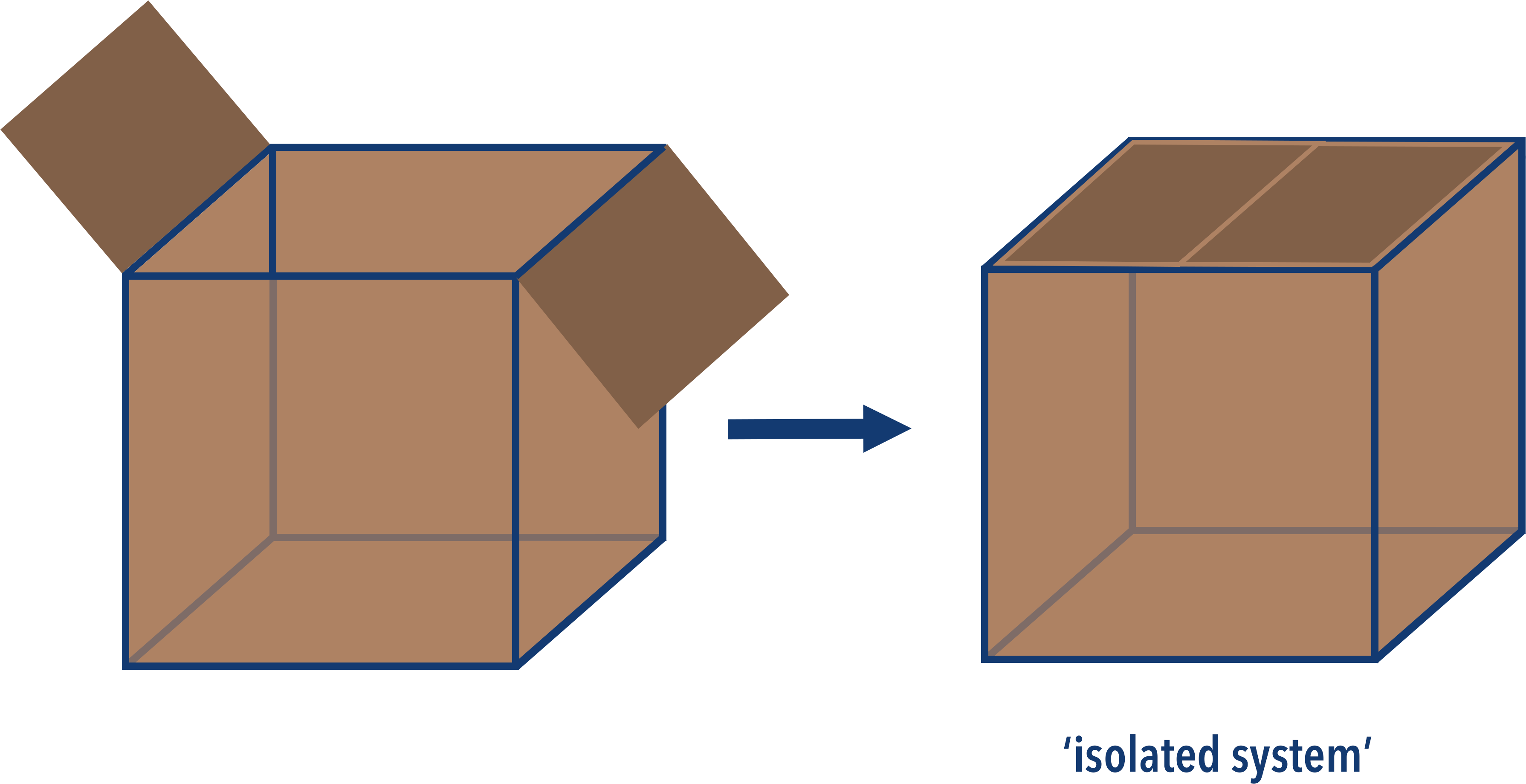If nothing is allowed to get in or out of the box (including heat) then that means the total energy inside the box has to be constant - regardless of whatever happens inside it. In more advanced study, this would be referred to as an 'isolated system'. Note - in absolutely no way could a cardboard box EVER be an isolated system, the diagram is just to help visualise the idea. The only real true isolated system is the entire universe itself.

Inside this box (isolated system) there are the reacting particles and the surrounding particles. As the reaction occurs, the entropy of both of these will change (see above).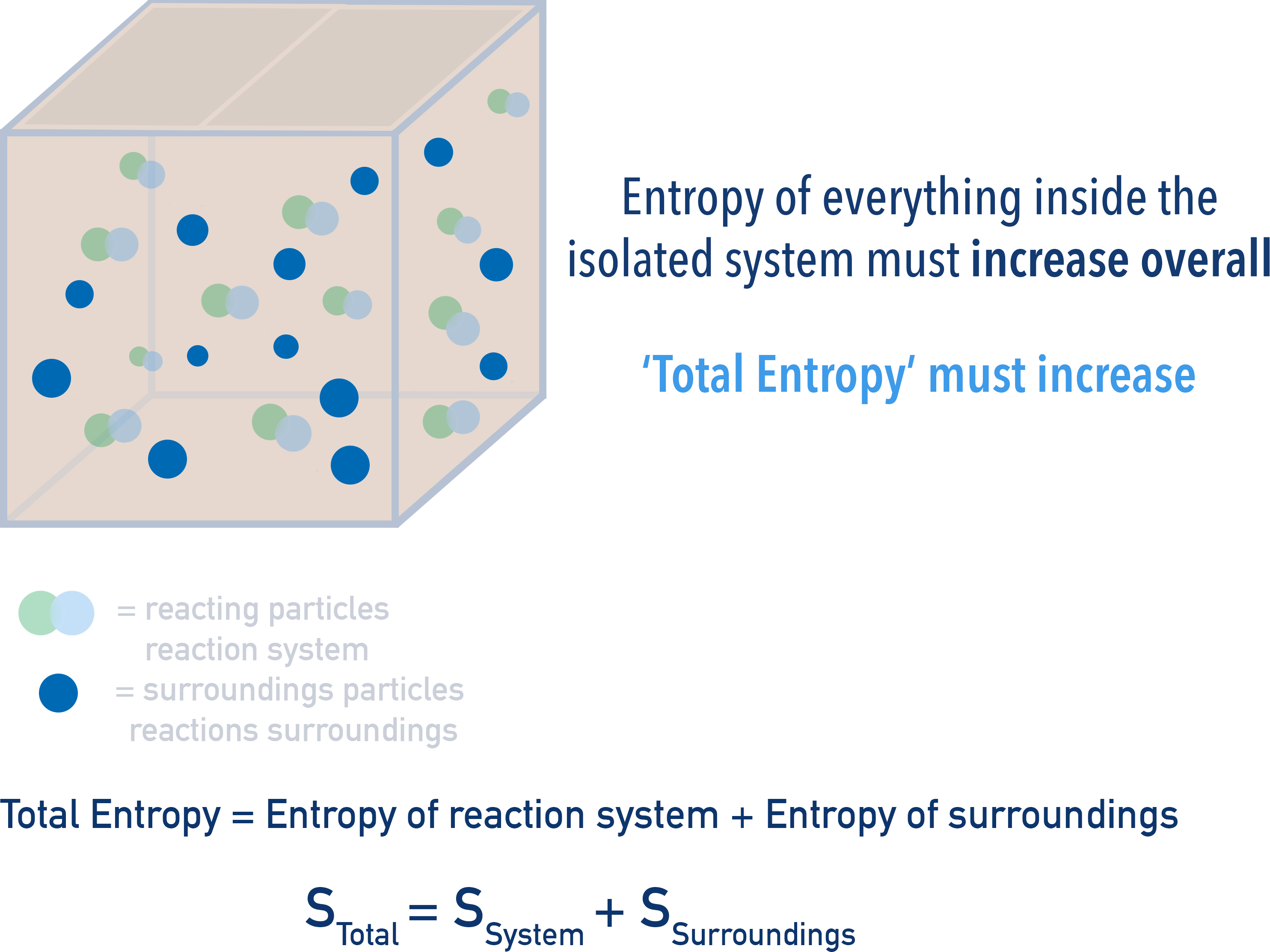Remember the total amount of energy in the box has to remain constant. This means the total entropy change that occurs is made up of both the change in entropy of reacting particles and their surroundings.

Even if the entropy change of the reaction is negative, as long as the entropy change of the surroundings is more positive, the overall total entropy change would be positive - meaning the reaction is considered feasible. The temperature of the surroundings has an impact on the entropy change of surroundings, meaning the temperature at which a reaction is carried out can determine whether it is feasible or not.